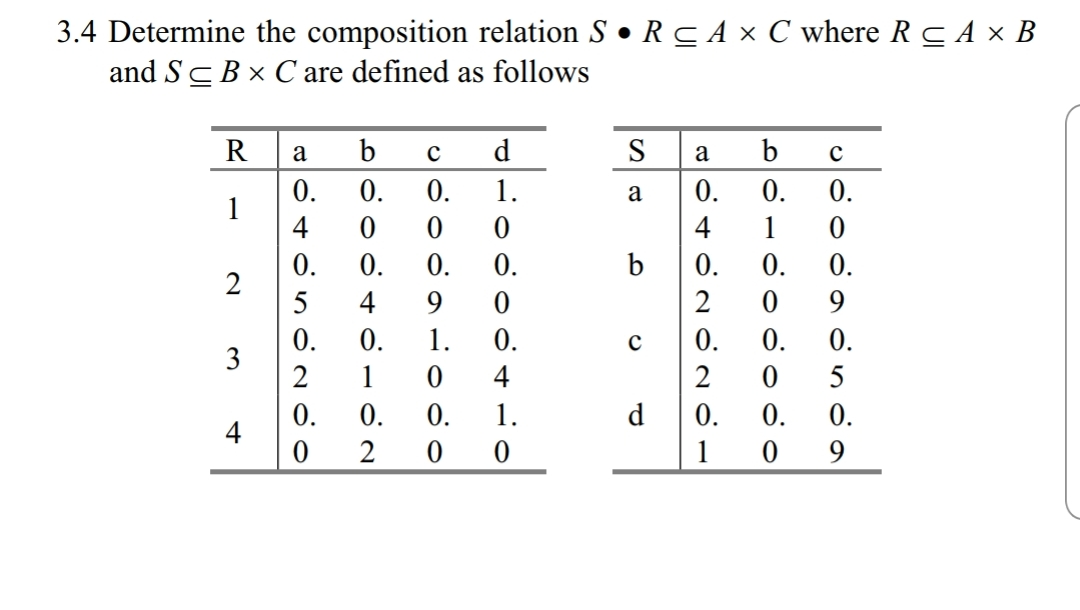3.4 Determine the composition relation S·R c A x C where Rand ş are defined as followsA × BR a bcdS a bc404 0 0 05 49 00 2 0 0

Question

the book is "First Course on Fuzzy Theory and Applications"help_outlineImage Transcriptionclose3.4 Determine the composition relation S·R c A x C where R and ş are defined as follows A × B R a bcd S a bc 4 0 4 0 0 0 5 49 0 0 2 0 0 fullscreen
Step 1

To  write down the table for the fuzzy composite relation

Step 2

Definition of the fuzzy composiition rule

Step 3

the table is completed following the formula given above. This ...

Want to see the full answer?

See Solution

Want to see this answer and more?

Our solutions are written by experts, many with advanced degrees, and available 24/7

See Solution
Tagged in

Math